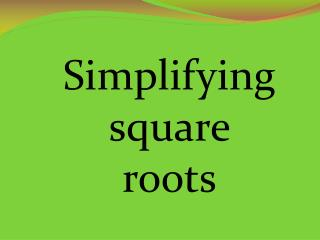# Simplifying square roots - PowerPoint PPT PresentationDownload PresentationSimplifying square roots

Simplifying square rootsDownload Presentation## Simplifying square roots

- - - - - - - - - - - - - - - - - - - - - - - - - - - E N D - - - - - - - - - - - - - - - - - - - - - - - - - - -
##### Presentation Transcript

1. Simplifying square roots

2. Do these expressions have another exact representation of the value? Yes, the square root of 4 is also 2 because 2 times 2 equals 4. Yes, the square root of 8 is also 2 times the square root of 2 or 2 square roots of 2 which is also written as 2 . No, the square root of 13 can not be renamed or simplified.

3. Standard: M8N1. Students will understand different representations of numbers including square roots, exponents, and scientific notation.g. Simplify, add, subtract, multiply, and divide expressions containing square roots. Essential Question: How do I simplify a square root?

4. If 92 = 81 then 9 is a square rootof 81 or = 9. In the expression , is the radical signand64 is the radicand.

5. Find the principle square root of 64. This is also written as . = 8 • Find the negative square root of 0.04. This is also written as : . [reminder: .04 is 4 hundredths; the square root of 4 is 2; the square root of one hundredth is one tenth] = - 0.2

6. 3. Find the square roots: 11, -11 This can also be written ±11. 4. Find the square root: 21 5. Find the square root:

7. What numbers are squares? 1 x 1 = 1 2 x 2 = 4 3 x 3 = 9 4 x 4 = 16 5 x 5 = 25 6 x 6 = 36 49, 64, 81, 100, 121, 144, ...

8. 1. Simplify Factor 80. 80 = 2 x 2 x 2 x 2 x 5 Now, you may “take out” pairs of like factors = You did it! =

9. 2. Simplify Factoring 180 into prime factors you get 2 x 2 x 3 x 3 x 5. Yes! Good job!

10. Now, try one by yourself. Simplify and see if your answer is below. Did you get it? Congratulations!!! 1. . 2. . 3. . 4..

11. 3. Simplify Simplify the numerator and denominator.

12. 4. Simplify Simplify the numerator and denominator. You could also solve this problem by dividing 100 by 25 first. Let’s see that. Cool, huh? Both ways work so you have a choice.

13. Please work these problems showing all steps. 1. 2. 3. 4. 1. 2. 3. 4. Anything we need to go back over before we continue?

14. Good job! Now, work these problems showing all steps. 5. 6. 7. 8. 5. 6. 7. 8.

15. How do I simplify a square root? Now, practice by completing your worksheet.